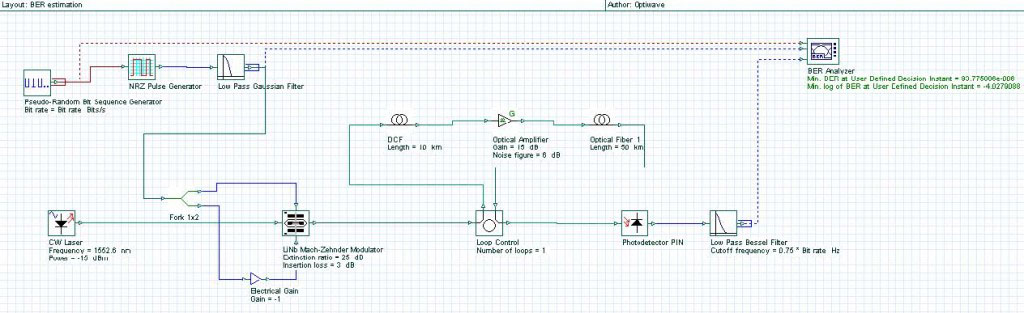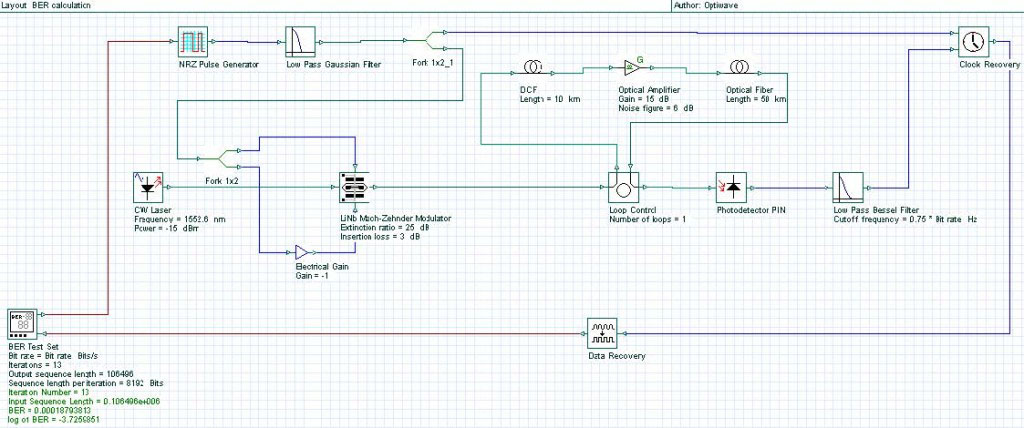This example demonstrates the use of a BER test set component to calculate the system performance.

Samples:

NRZ_BERestimation.osd

NRZ_BERcalculation.osd

Fig. 1 shows the system layout designed that will have its performance evaluated. For an initial estimation on the BER value, a BER analyzer is used to estimate the bit error rate based on a Gaussian algorithm with transmission of a short sequence of bits.Figure 1: System layout with BER estimated

For the BER calculation using the BER test set, the number of errors is counted and therefore the number of bits transmitted has to be large enough to obtain the BER value estimated. Changes were made in the last layout to set up the system to count the number of errors:

–     By using the system already designed, replace the Pseudo-Random Bit

Sequence Generator component by the BER Test Set component;

–     After the electrical Low Pass Bessel Filter, introduce the Clock Recovery component to synchronize the input and output signals;

–     After Clock Recovery component, introduce a Data Recovery component to convert the electrical signal to binary data;

–     In the global parameters, set the sequence length that will be transmitted in each block (e.g., 8192 in this example);

–     In the BER Test Set component

o    enable the parameter Truncate sequence;

o    set the Sequence length parameter, in the last example the BER estimated was around 1e-4, then any sequence length longer than 100k should be ok;

o    update the Order parameter to cover the sequence length used;

o    once you click Ok in the Data Recovery component, the Iterations parameter will be updated. Get this value (in this example it is 13) and set the Iterations global parameter (signals tab) at this value.

–     Before run the system, we need to adjust the parameters for the Data

Recovery component

o    Go to the BER Test Set component and in the Enhanced tab enable the

Single iteration parameter;

o    Introduce a electrical oscilloscope visualizer at the output port of the clock recovery component;

o    Run the system;

o    After that go to the Oscilloscope and check in the signal graph which values of threshold and instant decision would be reasonable to use (in the example, it was 8µ a.u and 0.5 bit);

o    Based on these values go to the Data Recovery component and set its parameters as demonstrated in the example.

–     After that you just have to disable the Single iteration parameter in the BER Test Set component and you can run the system.

After each iteration the BER value is updated and displayed by the BER test set.Figure 2: System layout with BER calculated